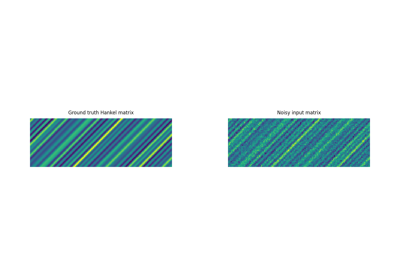# pyproximal.projection.HankelProj#

class pyproximal.projection.HankelProj[source]#

Hankel matrix projection.

Solves the least squares problem

$\min_{X\in\mathcal{H}} \|X-X_0\|_F^2$

where $$\mathcal{H}$$ is the set of Hankel matrices.

Notes

The solution to the above-mentioned least squares problem is given by a Hankel matrix, where the (constant) anti-diagonals are the average value along the corresponding anti-diagonals of the original matrix $$X_0$$.

Methods

 __init__(*args, **kwargs)

## Examples using pyproximal.projection.HankelProj#Hankel matrix estimation

Hankel matrix estimation